# 5-coloring graphs with small crossing & clique numbers (Solved)

 Importance: Medium ✭✭
 Author(s): Oporowski, Bogdan Zhao, David
 Subject: Graph Theory » Topological Graph Theory » » Coloring
 Keywords: coloring crossing number planar graph
 Posted by: mdevos on: October 9th, 2007
 Solved by: R. Erman, F. Havet, B. Lidicky and O. Pangrac, 5-colouring graphs with 4 crossings, in preparation.

For a graph, we let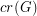denote the crossing number of, and we letdenote the size of the largest complete subgraph of.

Question   Does every graphwithand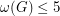have a 5-coloring?

In a nifty paper [OZ], Oporowski and Zhao get a little more mileage out of the classic Kempe-chain proof of the 5-color theorem, proving the following result.

Theorem  (Oporowski and Zhao)   Every graphwithandis 5-colorable.

In particular, this shows that every graph with crossing number at most 2 is 5-colorable. The graph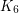can be drawn in the plane with three crossings, so it is clear that the assumptionis necessary in the highlighted theorem. However, it is possible that the assumptionmight be relaxed. The most extreme example known is the graphobtained fromby removing the edges of a 5-cycle. This graph has,and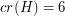, showing that thebound in the question is best possible.

Erman, Havet, Lidicky and Pangrac [EHLP] further improve this to

Theorem  (Erman et al.)   Every graphwith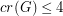andis 5-colorable.

On the other hand, the graph with,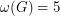and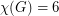(disproving the original conjecture) can be constructed in the following way: take two copies of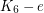with non-edges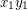and, respectively. Let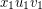and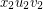be two triangles in these copies, and identify the corresponding vertices in these triangles. Finally, add the edge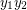.

## Bibliography

*[OZ] B. Oporowski and D. Zhao, Coloring graphs with crossings.

[EHLP] R. Erman, F. Havet, B. Lidicky and O. Pangrac, 5-colouring graphs with 4 crossings, in preparation.

* indicates original appearance(s) of problem.